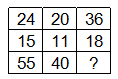Question 16

# Select the missing numbers from the given alternativesSolution

The pattern followed is that in the first row, the number in the middle is obtained by adding the other two and dividing the result by 3.

Eg : $$\frac{24+36}{3}=\frac{60}{3}=20$$

and $$\frac{15+18}{3}=\frac{33}{3}=11$$

Similarly, $$\frac{55+x}{3}=40$$

=> $$x=120-55=65$$

=> Ans - (D)

• Free SSC Study Material - 18000 Questions
• 230+ SSC previous papers with solutions PDF